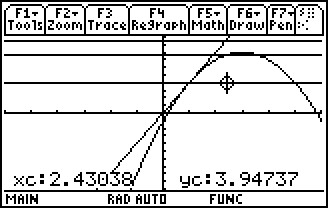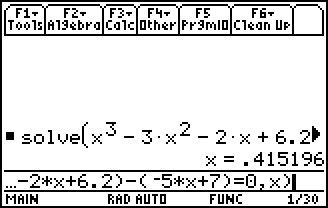# Activities

••• ##### Subject Area

• Math: Calculus: Applications of the Derivative

• ##### Author9-12

45 Minutes

• ##### Device
• TI-89 / TI-89 Titanium

## Linear Approximation - 2#### Activity Overview

Students graph the functions, construct the tangent line at a point, and find an estimate from a linear approximation.

#### Key Steps

•Students will graph the functions, construct the tangent line at a point, and find an estimate from a linear approximation.

•Students will then determine whether the estimate is an overestimate or an underestimate, and find an interval for a desired accuracy.